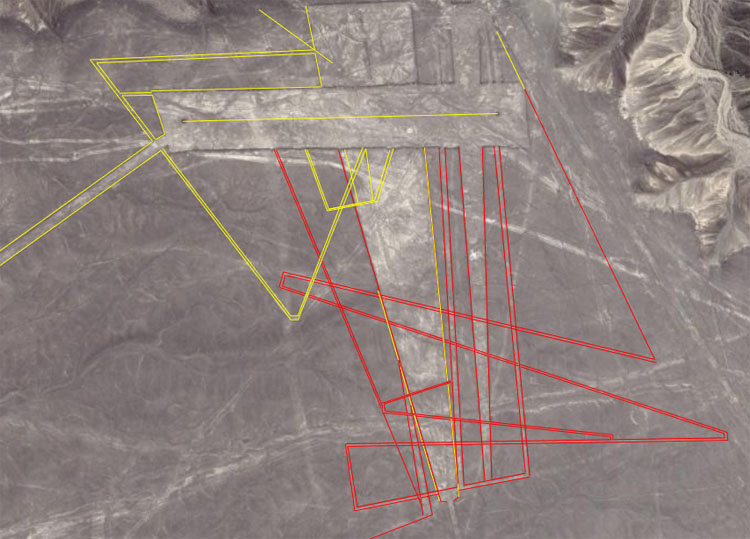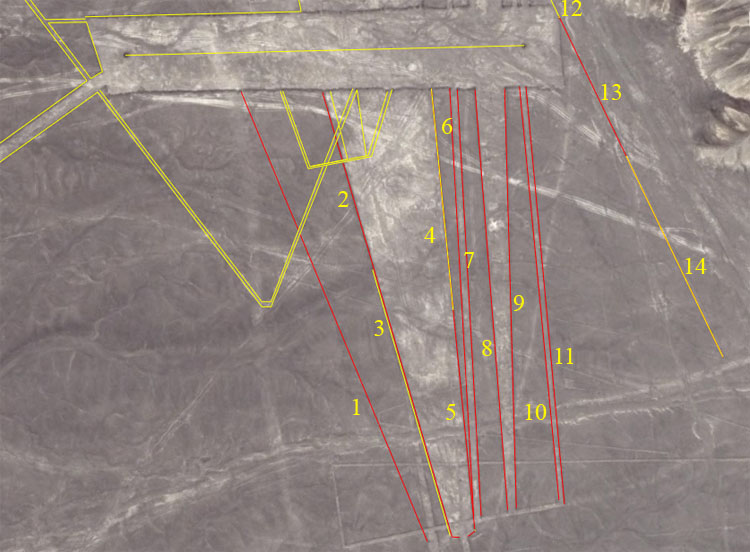TRAPEZOID AND MISCELLANEOUS CODES.We'll now move to the trapezoids or appendage geometry that branches off or through them. The area of discussion will be, primarily, the red lines seen above. For visual ease, this maze of lines will be numbered in two parts. First the lines closer to the vertical.1. The distance is 1320-feet and the angle is 337.5-degrees.

The value of 1320-feet is 1/4th of a mile of 5280 feet,

The degree angle of 337.5-degrees is both lunar and navigational and a mathematical progression based upon this number produces a string of values very useful to both. The main cycle numbers of 6804-days and 2551.5-days produce navigational numbers when divided by 3.375 (6804 ÷ 3.375 = 2016 ... or 2 X 100.8-feet ...1 second of arc under the Great Pyramid's equatorial circumference was 100.8-feet). Alternatively 2551.5 ÷ 3.375 = 756 ... the length of the Great Pyramid or 1/8th of 1-minute of arc.

2. The distance is 504-feet and the angle is 343.75-degrees.

The architects who designed this long line at the side of the trapezoid were very clever and broke it into two main parts, each carrying a dynamic code. The length of this part is 504-feet and a mathematical progression based upon this value produces excellent navigational values. Twice 504 = 1008, meaning that this length is 5-seconds of equatorial arc.

The degree angle contains navigational codes that pertain to the mile of 5280-feet on the equatorial circumference of 24750-miles. There would be 72 increments of 343.75-miles in the 24750-mile circumference.

3. The second part of the trapezoid side extends for 756-feet, or the length of the Great Pyramid. The angle remains 343.75-degrees.

The designers of the line down the side of the trapezoid cleverly combined the two halves to achieve a dynamic overall code (504 + 756 = 1260). The length of 1260-feet is 120 Hebrew Reeds or two Greek stadias. It is also 1296 Roman feet (remember, the Precession of the Equinoxes endures for 25920-years and 1296-years is half that period).

4. Again, down the opposite side of the trapezoid are multiple codes at various stations. This length is 560-feet and the angle is 354.375-degrees.

5. The overall distance is 1209.6-feet, which is a navigational code and the angle remains 354.375-degrees.

The 1209.6-feet code is navigational. The value of one circuit of the Great Pyramid's perimeter is 3024-feet or 36288-inches (2 X 12096). Under the Great Pyramid's assignment for the equatorial circumference of the world, 1-degree of arc was 362880-feet or 120960 X 2. This distance at Nazca was 1/108000th of the equatorial circumference.

The 354.375-degree angle is in homage to the 354.375-days in the lunar year.

6. The distance is 1166.66666 and the angle is 176.7857143-degrees (176 & 11/14ths).

This distance is simply 1200 Roman feet and would represent 1/112500th of the equatorial circumference of the Earth under the Roman system.

Another code that would have been read into this distance is 1181.25-feet, if the line is extended across the horizontally dissecting pathway to the other side. The period of 1181.25-days would be 40 lunar months.

The angle code is an expression of dividing the 24750-mile equatorial circumference into 7th values and 176 & 11/14ths-miles would be 1/140th of the circumference under that system.

It might be said at this point that the ancient mathematician-astronomers had an almost obsessive-compulsive fixation on numbers and how to manipulate them. They used numbers in every conceivable category and from these formulated mathematical progressions that could branch from a main line to appendage lines... in much the same vein as they made their quippu knot calculators with their secondary lines branching off main pendants. In some respects the mathematical progressions could be compared to chord families in music, where the artist is playing in one key, but can immediately jump back and forth to related keys without producing disjointed notes that grate on the ear of the listener. The values used in all of the progressions are merely harmonic numbers in sequences. A good jazz improvisor who has studied the science of harmony deeply and memorised the family relationships would understand this concept empirically.

7. The distance and angle remain 1166.666666-feet & 176 & 11/14ths-degrees.

The only interruption to this is found where crossing lines traverse the main line. One secondary code is 672-feet and this value was very important to ancient navigation. A progression based upon this goes 672, 1344, 2016, 2688, 3360, 4032, 4704, 5376, 6048 (note: 1-minute of equatorial arc was 6048-feet under the Great Pyramid's assignment), etc. Another station further down gives a reading of 787.5-feet, which is part of a progression that produces both navigational and lunar numbers.

8. The distance is 1152-feet and the angle is 355.55555-degrees (355 & 5/9ths).

The 1152 value is navigational

The angle is 3200 ÷ 9.

9. The distance is 1145.833333 (1145 & 5/6ths) and the angle is 358.4-degrees.

The 1145 & 5/6ths value is navigational and under the 24750-mile equatorial circumference 114.5833333-miles would be 1/216th (a Precession of the Equinoxes value).

The angle is useful as a conversion ratio for turning the Great Pyramid equatorial circumference figure (24883.2 Greek miles or 130636800 standard feet into Roman or lunar values. Thus 130636800 ÷ 358.4 = 3645. There would be 16 increments of 3.645-inches in the Roman lunar Pace of 58.32-inches. The raw value of 358.4 is essentially navigational.

10. The distance is 1134-feet and the angle is 354.53333-degrees (354 & 8/15ths).

The distance is a very dynamic lunar code,

The angle, one would expect, should have been 354.375 or .1583333 of 1-degree less. When viewing this angle 180-degrees opposed it equates to 174.53333 and seems to be a part of a PI progression. The value of PI, in enlarged numbers, could be represented as 3141.6, which is 174.53333 X 18. We must remember that many different students and masters of varying talent or observation skills were creating these lines with manual instruments. It should be expected that. occasionally, some detectable drift will show up, just as it does in the work of modern day surveyors. It is the opinion of this researcher that the intended angle was 354.375-degrees.

11. The distance is 1145.833333-feet (1145 & 5/6ths) and the angle remains the same at 174 & 8/15ths.

The length carries a navigational code that relates to the equatorial circumference under the 24750-mile system.

12, 13 & 14. A barely detectable line runs for a considerable distance, on the diagonal, to the eastern side of the site. It appears to be divided into at least 3 sections. At the top section the line smudges out and it's a little difficult to clearly define the termination point. It appears that the line is segmented into several codes, which include (a) 1260-feet or 120 Hebrew Reeds; (b) 1244.16-feet to code half of 2488.32-feet; (c) a segment representing 1050-feet coupled to a segment of 210-feet (1260 total); (d) a probable segment representing 1031,25-feet to code the "11" family Egyptian Royal Cubit of 20.625-inches. The length of 1031.25-feet would be 600 cubits.

The angle is based upon 7th divisions of the navigational value 1080. The angle is 154.2857143-degrees (154 & 2/7ths) and this value would be 1/7th of 1080. As it turns out, there is an exposed ancient wall at Cahuachi pyramid complex 9-miles to the SSE of this site of the Nazca desert site that we're studying, which has a length of 1080-feet and its angle off north is 96-degrees.

Continue